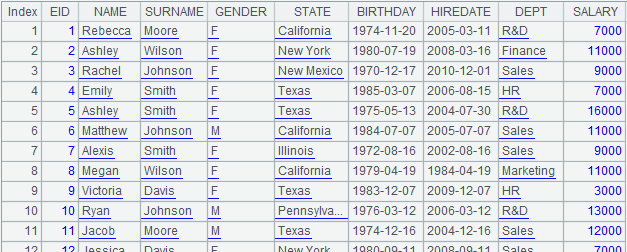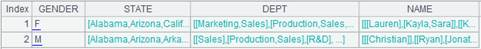• 集算器
教程
函数参考
习题
代码参考
用户参考
外部库使用指南
数据文件工具用户参考
DQL教程
集群管理器使用说明
SPL命令执行器用户参考
• 润乾报表
教程
填报教程
分析教程
报表中心教程
用户参考
程序员参考
高级指南
通用查询
DQL部署集成手册
• 易明建模
用户参考
json参数说明
• 润乾官网

# index()

## T.index()

T.index(n)

 T 有键的序表 n 索引长度

 @s T的基本键是排号时则建立成多层树状索引，忽略n @m 并行建立 @n 为序表建立序号索引；序号索引用于外键序号化，要求事实表的外键值对应维表记录的序号，使用时序号键可省略；使用该选项时将忽略参数n； 该选项不适用于序表中有时间键的情况

 A 1 =demo.query("select EID,NAME,SALARY from EMPLOYEE where EID<4") 2 =A1.keys(EID) 设置A1的键为EID 3 =A1.index(10) 为序表键建立长度为10的索引表 4 =A1.index@m(100) 并行建立索引表

 A 1 =connect("demo").query("SELECT * FROM CITIES") 返回序表2 =connect("demo").query("SELECT * FROM STATECAPITAL").keys(STATEID) 返回序表，并设置序表的键为EID3 =A2.index@n() 为A2表建立序号索引 4 =A1.switch(STATEID,A3) CITIES表作为事实表，STATECAPITAL作为维表，将CITIES表中的STATEID键值切换为STATECAPITAL的指引记录，计算过程中会使用外键STATEID的索引表，此时表达式中可以省略序号键STATEID，等同于A1.switch(STATEID,A3:#)

## T.index(n)

T.index(n)

 T 主键唯一的内表 n 索引长度。

 @m 并行建立 @n 可用序号键建立索引，序号键可省略，但有时间键时不能省略 @s 可用排号键建立索引

 A 1 =file("D:\\emp3.ctx") 2 =A1.create(#EID,NAME) 创建组表基表 3 =demo.cursor("select EID,NAME from employee where EID< 10") 4 =A2.append(A3) 5 =A2.attach(table3,#GENDER) 在基表上添加附表 6 =demo.cursor("select EID,GENDER from employee where EID< 10") 返回游标 7 =A5.append(A6) 在附表中追加游标记录 8 =A5.memory() 用附表生成内表 9 =A8.keys(EID) 设置内表的键为EID 10 =A8.index(10) 建立长度为10的索引表 12 =demo.cursor("select * from employee").memory() 返回内表 13 =A12.keys@t(EID,HIREDATE) 设置内表的基本键为EID，时间键为HIREDATE 14 =A12.index@n() 为内表建立索引

## T.index(I:h,w;C,…;F,…)

T.index(I:h,w;C,…;F,…)

h时创建平均长度为hhash索引；创建索引时必须存在列C和索引名称I，索引名称I不可重复，索引创建成功后自动生成名称唯一的索引文件。

F为实表的字段名，有参数F时，将F字段记入索引文件，当使用该索引取出记录的时候，只能取出索引字段C和字段F，该类索引的优势在于可以通过C的值快速查询出来F的值。F省略时索引中记录所有实表字段的位置信息。

 T 实表/复组表 I 索引名称 w 筛选条件，可省略，缺省读取全集 C 建立索引的字段 h 索引长度 F 实表的字段名，可省略

 @2 只有参数I时，主动加载索引的第二层并保持 @3 只有参数I时，主动加载索引的第三层并保持 @0 只有参数I时，释放 @w 按列C建立全文检索，可支持like(“*X*”)式检索

 A 1 =file("emp1.ctx") 2 =A1.create(#EID,NAME) 创建组表基表 3 =demo.cursor("select EID,NAME from employee where EID< 10") 4 =A2.append(A3) 在基表中追加游标记录 5 =A2.attach(table1,DEPT,GENDER) 在基表上增加附表table1 6 =demo.cursor("select EID,DEPT,GENDER from employee where EID< 10") 7 =A5.append(A6) 在附表table1中追加游标记录 8 =A7.index(test_index1,GENDER=="F";DEPT;) 用DEPT字段建test_index1索引，索引文件名称为：emp1.ctx_table1_test_index1 9 =A5.index(test_index3,["F"].contain(GENDER);DEPT;) 使用contain语法，用DEPT字段建test_index3索引，索引文件名称为：emp1.ctx_table1_test_index3 10 =A5.index(idx1:10;DEPT;) 用DEPT字段创建长度为10的索引idx1 ，索引文件名称为：emp1.ctx_table1_idx1 11 =A5.index(test_index3) 删除索引test_index3 12 =A5.index() 删除所有索引 13 =A5.index(test_index,GENDER=="F";DEPT;GENDER) 建立的索引，在通过该索引取数时，只能取出索引字段DEPT和字段GENDER 14 =A7.index@w(test_index4,GENDER=="F";DEPT;) 按列DEPT建立全文索引 15 =A14.icursor (;like(DEPT,"*ale*"),test_index).fetch() 使用like(“*X*”)式查询16 =A5.index@2(test_index3) 主动加载索引的第二层并保持 17 =A5.index@3(test_index3) 主动加载索引的第三层并保持 18 =A5.index@0(test_index3) 释放索引

Ø  T为复组表：

 A 1 =file("test.ctx":[1,2,3]) 2 =A1.create@y(#EID,NAME,GENDER,DEPT;EID%3+1) 生成复组表 3 =connect("demo").cursor("select EID,NAME,GENDER,DEPT,GENDER from employee") 返回游标 4 =A2.append@x(A3) 在复组表中追加游标中的数据 5 =A4.index(test_index4,GENDER=="F";DEPT;) 每个分表创建一个索引## T.index(I:h,w;C,…)

T.index(I:h,w;C,…)

 T 内表 I 索引名称 w 筛选条件，缺省读取全集 C 建立索引的字段 h 索引长度

 A 1 =demo.cursor("select * from SCORES ") 2 =file("SCORES_ClassTwo.ctx") 创建组表文件 3 =A2.create@y(#CLASS,#STUDENTID,SUBJECT,SCORE) 创建组表的基表，其中CLASS,#STUDENTID为组表的键 4 =A3.append@i(A1) 将A1游标中的数据追加到基表中 5 =A4.memory() 生成内表 6 =A5.index(index1:10,CLASS =="Class one";SCORE) 对内表建立非主键索引